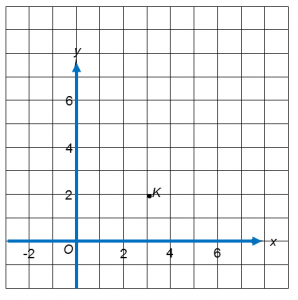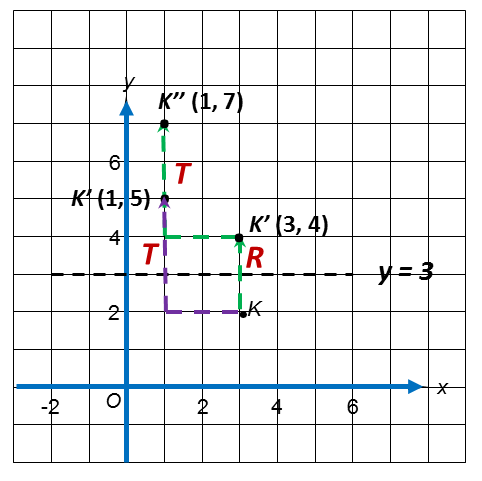# 5.5.5 Combined Transformation, SPM Paper (Long Questions)

Question 9:
(a)  Diagram 8.1 shows point K ( 3, 2 ) marked on a Cartesian plane.Diagram 8.1
The transformation T is a translation $\left(\begin{array}{l}-2\\ \text{}3\end{array}\right).$
The transformation R is a reflection in the line y = 3.

State the coordinates of the image of point K under each of the following transformations.
(i)   T
(ii)  TR [3 marks]

(b)  Diagram 8.2  shows three trapeziums, ABCD, PQRS and PTUV drawn on a Cartesian plane.Diagram 8.2

(i) Trapezium PTUV is the image of trapezium ABCD under the combined transformation VW
Describe in full, the transformation W and the transformation V.  [ 6 marks]
(ii) Given that the area of trapezium ABCD is 14 cm2, calculate the area, in cm2, of the shaded region. [3 marks]

Solution:(a)(i) (3, 2) → T → (1, 5)

(a)(ii)
(3, 2) → R → (3, 4) → T → (1, 7)

(b)(i)W : Rotation, 90o clockwise at centre (0 , −1)
V: Enlargement, scale factor of 2, centre of enlargement at point P (2 , 1)
(ii)
Area of PTUV = (scale factor)2 × Area of object
=  22  × 14
= 56 cm2
Area of shaded region = 56 – 14 = 42 cm2